Examples

Chapter 1 Class 11 Sets
Serial order wise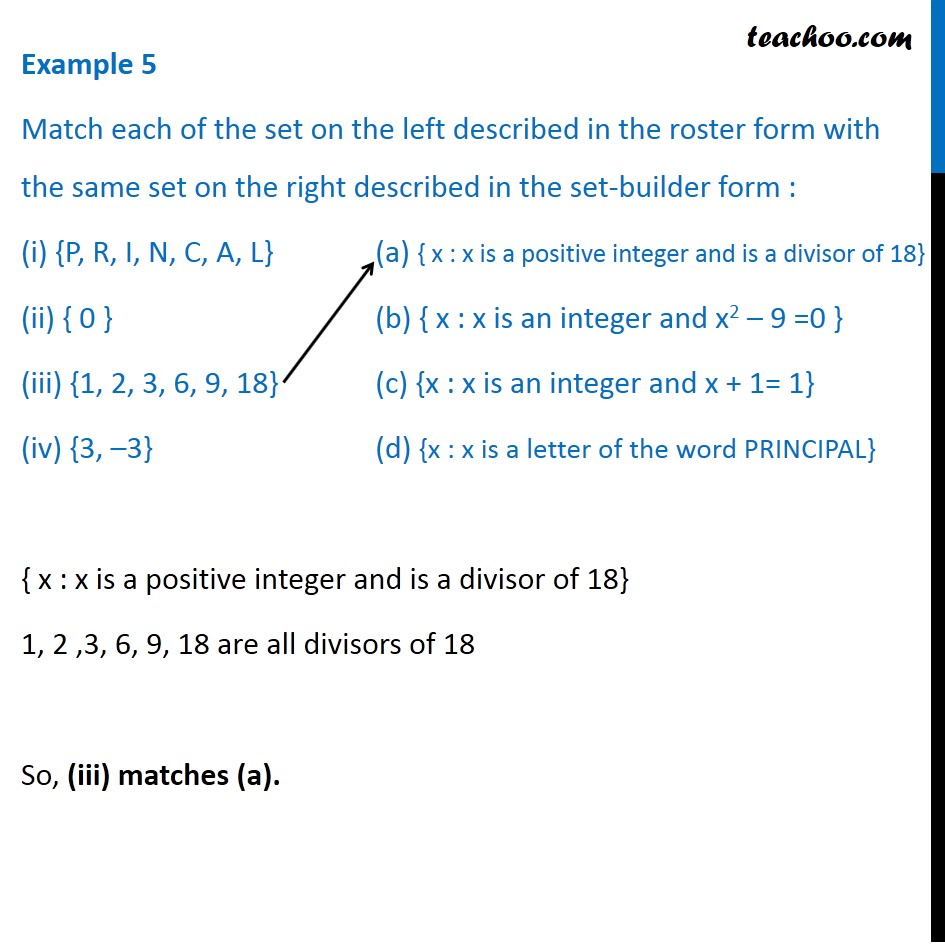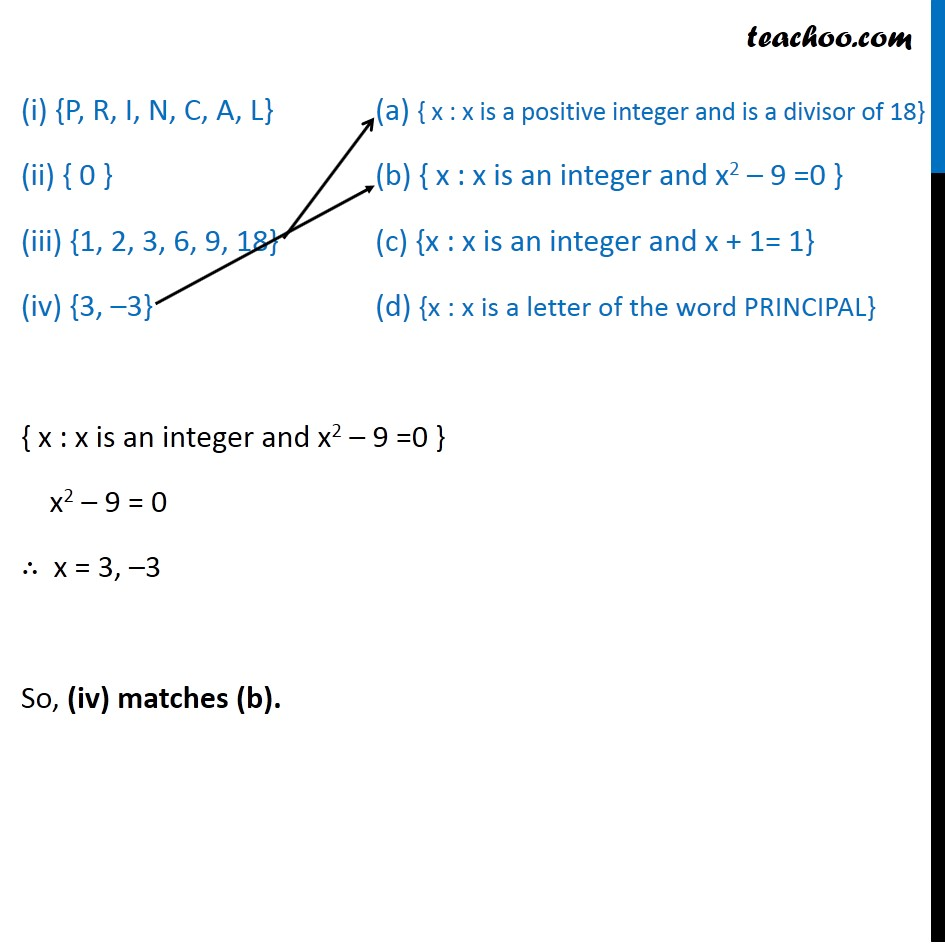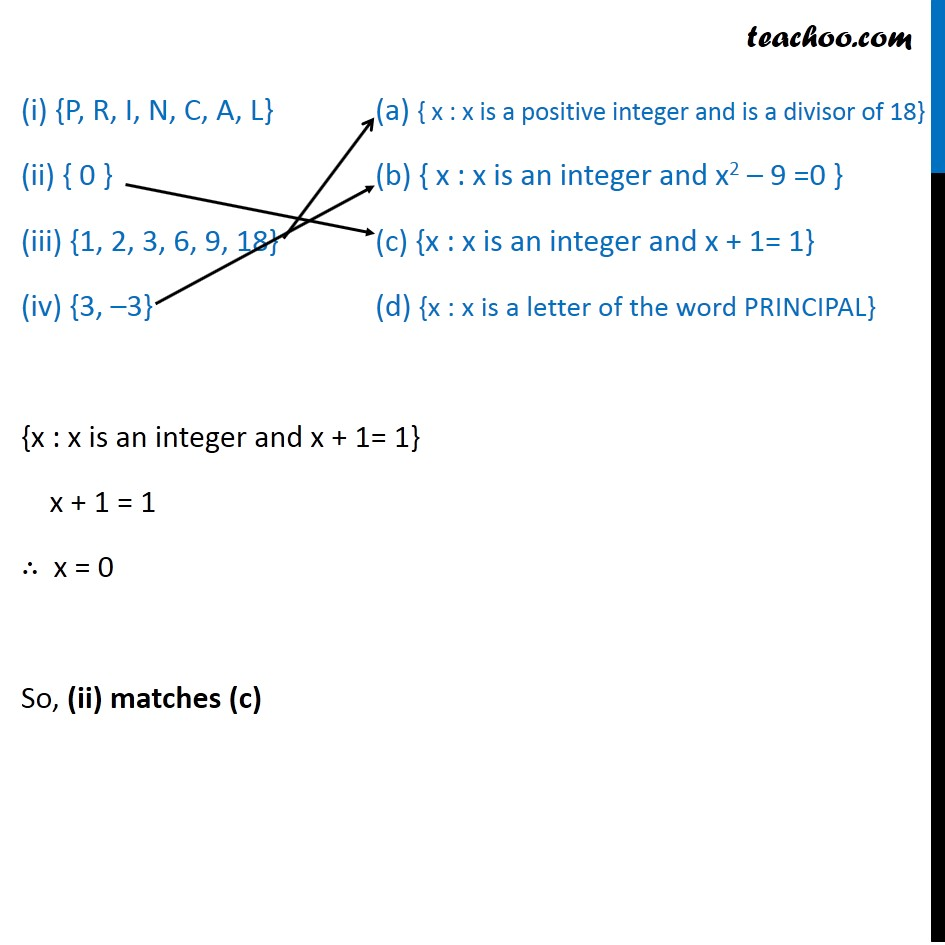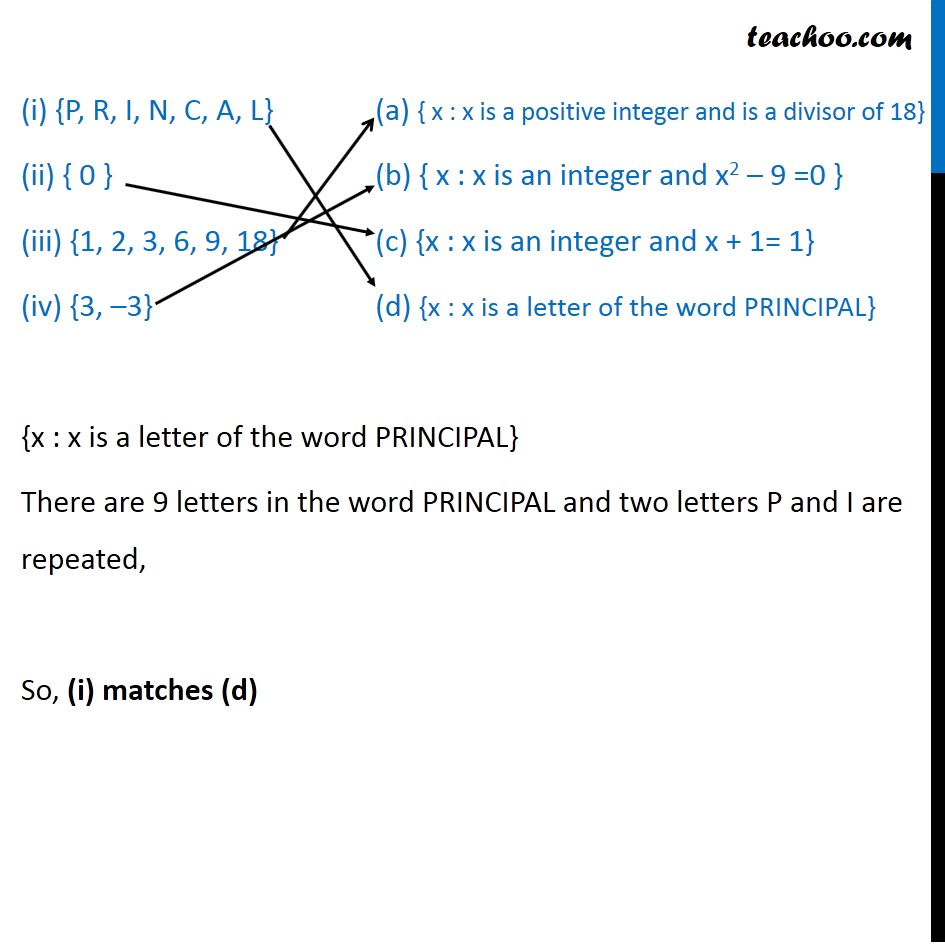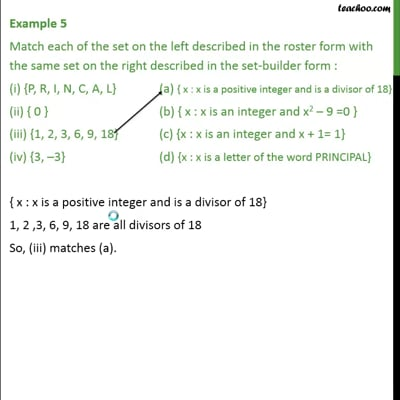This video is only available for Teachoo black users

Solve all your doubts with Teachoo Black (new monthly pack available now!)

### Transcript

Example 5 Match each of the set on the left described in the roster form with the same set on the right described in the set-builder form : (i) {P, R, I, N, C, A, L} (a) { x : x is a positive integer and is a divisor of 18} (ii) { 0 } (b) { x : x is an integer and x2 – 9 =0 } (iii) {1, 2, 3, 6, 9, 18} (c) {x : x is an integer and x + 1= 1} (iv) {3, –3} (d) {x : x is a letter of the word PRINCIPAL} { x : x is a positive integer and is a divisor of 18} 1, 2 ,3, 6, 9, 18 are all divisors of 18 So, (iii) matches (a). (i) {P, R, I, N, C, A, L} (a) { x : x is a positive integer and is a divisor of 18} (ii) { 0 } (b) { x : x is an integer and x2 – 9 =0 } (iii) {1, 2, 3, 6, 9, 18} (c) {x : x is an integer and x + 1= 1} (iv) {3, –3} (d) {x : x is a letter of the word PRINCIPAL} { x : x is an integer and x2 – 9 =0 } x2 – 9 = 0 ∴ x = 3, –3 So, (iv) matches (b). (i) {P, R, I, N, C, A, L} (a) { x : x is a positive integer and is a divisor of 18} (ii) { 0 } (b) { x : x is an integer and x2 – 9 =0 } (iii) {1, 2, 3, 6, 9, 18} (c) {x : x is an integer and x + 1= 1} (iv) {3, –3} (d) {x : x is a letter of the word PRINCIPAL} {x : x is an integer and x + 1= 1} x + 1 = 1 ∴ x = 0 So, (ii) matches (c) (i) {P, R, I, N, C, A, L} (a) { x : x is a positive integer and is a divisor of 18} (ii) { 0 } (b) { x : x is an integer and x2 – 9 =0 } (iii) {1, 2, 3, 6, 9, 18} (c) {x : x is an integer and x + 1= 1} (iv) {3, –3} (d) {x : x is a letter of the word PRINCIPAL} {x : x is a letter of the word PRINCIPAL} There are 9 letters in the word PRINCIPAL and two letters P and I are repeated, So, (i) matches (d)